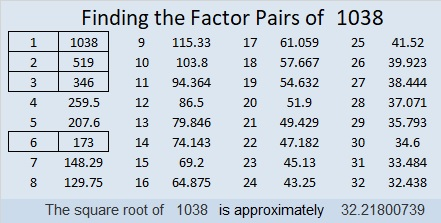# 1038 Hoppy Easter/April Fool’s Day

A trickster Easter bunny left some of my grandchildren candy and other treasures, not in a traditional Easter basket but in pink, green, and orange pumpkins! It’s April Fool’s Day so we shouldn’t be surprised.

That same trickster bunny has a purple pumpkin puzzle for YOU to try today, too. Part of the puzzle is easy while other parts are tricky: Is it 3 or 5 that is the common factor of 30 and 15 that makes this puzzle work?

Hmm…can you figure out where to put the numbers 1 to 10 in the first column and the top row or will you be tricked this April Fool’s Day?Print the puzzles or type the solution in this excel file: 10-factors-1035-1043

Now I’ll tell you a little bit about the number 1038.

1 + 8 is divisible by 3 so 1038 is also divisible by 3. (Including multiples of 3 in the sum isn’t necessary for that divisibility trick to work.)

• 1038 is a composite number.
• Prime factorization: 1038 = 2 × 3 × 173
• The exponents in the prime factorization are 1, 1, and 1. Adding one to each and multiplying we get (1 + 1)(1 + 1)(1 + 1) = 2 × 2 × 2 = 8. Therefore 1038 has exactly 8 factors.
• Factors of 1038: 1, 2, 3, 6, 173, 346, 519, 1038
• Factor pairs: 1038 = 1 × 1038, 2 × 519, 3 × 346, or 6 × 173
• 1038 has no square factors that allow its square root to be simplified. √1038 ≈ 32.2180071038 is also the hypotenuse of a Pythagorean triple:
312-990-1038 which is 6 times (52-165-173)

This site uses Akismet to reduce spam. Learn how your comment data is processed.